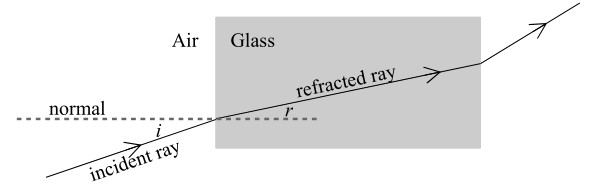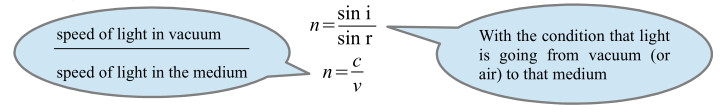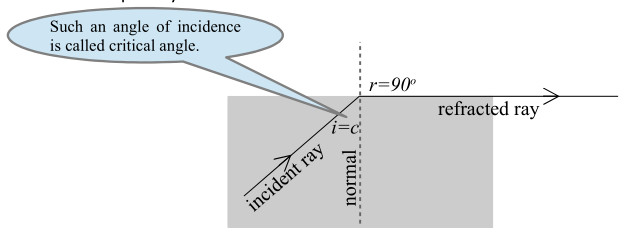Home » Handbook of O Level Physics » UNIT 14: REFLECTION AND REFRACTION OF LIGHT

# UNIT 14: REFLECTION AND REFRACTION OF LIGHT

• Beam: It is a bundle of rays.

• Shadows: They are the dark areas that are formed when light is blocked.

• We can see

• luminous objects because they produce light

• non-luminous objects when they reflect light

• Regular and irregular reflections

• Smooth, shiny and polished surfaces (e.g., mirror) give a regular reflection.

• Rough, dull and unpolished surfaces (e.g., paper) give an irregular reflection.

• Second Law of Reflection: The angle of incidence is equal to the angle of reflection.

i = r

• Plane mirrors

• Characteristics of image in a plane mirror

• Same size as the object.

• Same distance behind the mirror as the object in front of the mirror.

• Upright

• Virtual

• Laterally inverted ( i.e., left appears right and vice versa.)

• Applications of plane mirrors

• Eye-sight testing in a small room

• Removing parallax error in instrument scales

• Real Image: It is an image that can be formed on a screen because rays converge on image.

• Examples: Projector / Photocopier / Camera

• Virtual Image: It is an image that cannot be formed on a screen because rays only appear to come from image.

• Examples: Magnifying glass / Reflection in a mirror

• Refraction: It is the bending of light when it enters or exits a medium.

• When light slows down it bends towards the normal………….when light speeds up it bends away from the normal.

• Second Law of Refraction: The ratio of the sine of the angle of incidence in one medium to the sine of the corresponding angle of refraction in the other medium is a constant value.• Refractive index (of a medium): It is the ratio of the sine of the angle of incidence in vacuum (or air) to the sine of angle of refraction in the medium.• You use the formula n = (sin i / sin r) when light goes from vacuum (or air) to an optically dense medium
• You use the formula (1/n) = (sin i / sin r) when light goes from an optically dense medium to vacuum (or air)
• Value of n is always greater than 1.
• Greater the value of n, greater is the refraction (bending).
• Consequences of refraction

• Swimming pools appear shallower.

• Objects appear bent.

• Mirages

• Rainbows

Note: Rainbows are due to refraction, total internal reflection and dispersion of white light.

• Critical angle: It is the angle of incidence in the optically denser medium for which the angle of refraction in the optically less dense medium is 90o.• Total internal reflection: All the ray reflects back into the denser medium when the angle of incidence in the optically denser medium is greater than the critical angle.

• Application in fibre optic cables: Information is passed in the form of (visible) light that shows total internal reflection. Optical fibers are used in telecommunications because they have following advantages over copper wires:

• more data per second

• more secure

• less attenuation (so boosters are further apart)

• less interference (e.g., cross-talk)